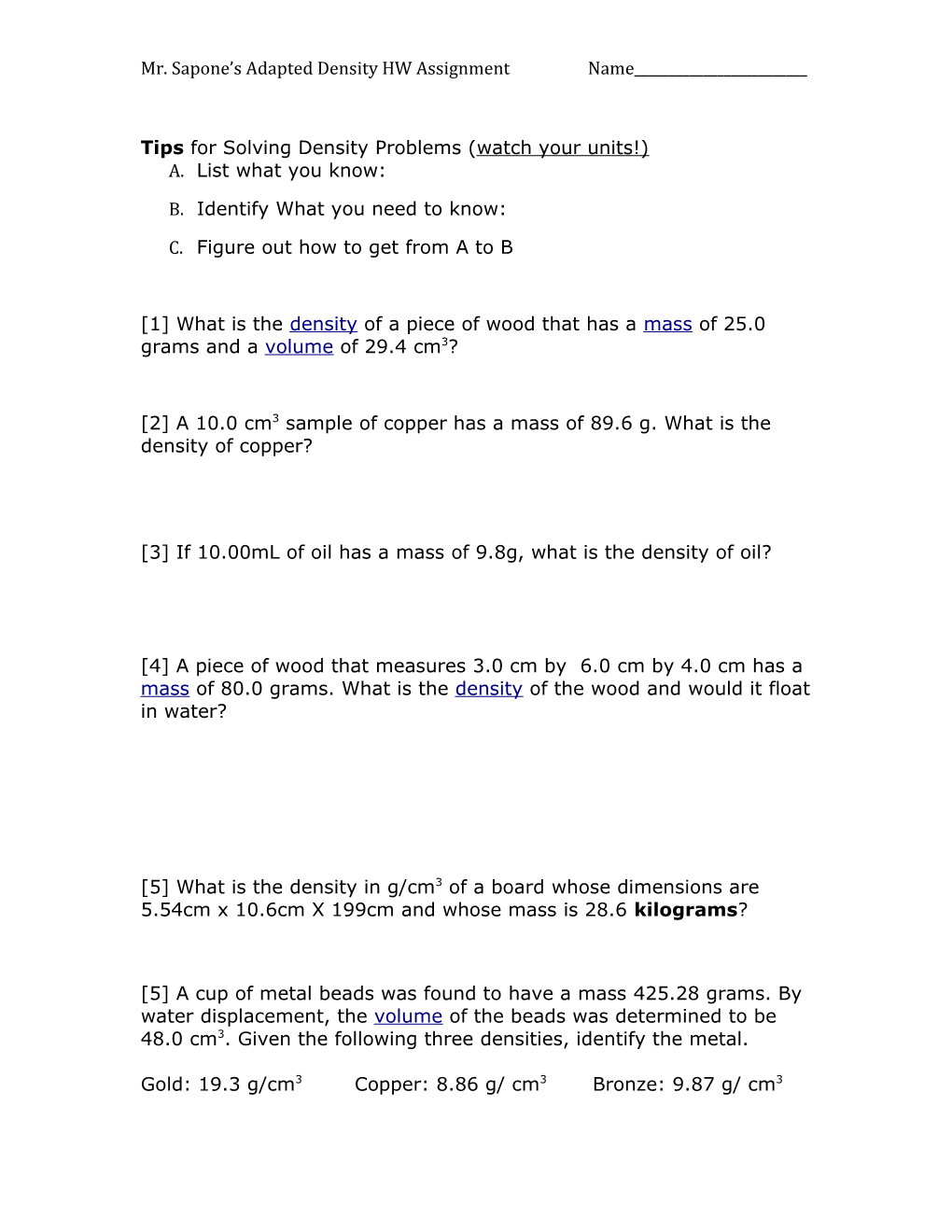# Mr. Sapone Sadapted Density HW Assignment Name______Mr. Sapone’sAdapted Density HW Assignment Name______

Tips for Solving Density Problems (watch your units!)

1. List what you know:
2. Identify What you need to know:
3. Figure out how to get from A to B

 What is the density of a piece of wood that has a mass of 25.0 grams and a volume of 29.4 cm3?

 A 10.0 cm3 sample of copper has a mass of 89.6 g. What is the density of copper?

 If 10.00mL of oil has a mass of 9.8g, what is the density of oil?

 Apiece of wood that measures 3.0 cm by 6.0 cm by 4.0 cm has a mass of 80.0 grams.What is the density of the wood and would it float in water?

 What is the density in g/cm3 of a board whose dimensions are 5.54cm x 10.6cm X 199cm and whose mass is 28.6kilograms?

 A cup of metal beads was found to have a mass425.28 grams.By water displacement, the volume of the beads was determined to be 48.0 cm3.Given the following three densities, identify the metal.

Gold: 19.3 g/cm3 Copper:8.86 g/ cm3 Bronze:9.87 g/ cm3

 If you had two cubes with the same mass, the cube with a higher volume will be (more/less) dense than the other cube.

 If you had two cubes with the same volume, the cube with a smaller mass will be (more/less) dense than the other cube.

 If an object has a mass value (in grams) that is less than its volume (in cm3) it will have a density value that is ( >, < or = ) 1 and will most likely (sink/float).

 If an object has a mass value (in grams) that is greater than its volume (in cm3) it will have a density value that is ( >, < or = ) 1 and will most likely (sink/float).

A plastic ball has amass of 125 grams.What must the volume be if it has a density of 0.500 g/cm3.

 Gold has a density of 19.3g/cm3. How many grams would 25cm3 of gold be?

 Iron has a density of 7.8g/cm3. If you had 445g of iron, how much space would it take up?

Calculate the density of sulfuric acid if 35.4 mL of the acid weighs 65.14 g

 What volume of silver metal will weigh exactly 2500.0 g. The density of silver is 10.5 g/cm3.

 If the density of 45.0 cm3 block of wood is 0.65 g/ml calculate the wood’s mass.

 A bar of chocolate is cut in half, what happens to its density?

 An irregularly shaped stone was lowered into a graduated cylinder holding a volume of water equal to 14.0 ml. The height of the water rose to 19.0 ml. If the mass of the stone was 28.0 g, what was its density?

 If a peach has a volume of 130 cm3and sinks in water, what can you say about its mass?

 If a banana has a mass of 150 grams and floats in water, what can you say about its volume?

 An empty cup has a mass of 50g. When you add water to it the mass increases to 86g. What is the volume of the water in the cup?

 How many cubic centimeters are there in a cubic meter?

 28.5 g of iron shot is added to a graduated cylinder containing 45.50 mL of water. The water level rises to the 49.10 mL mark, from this information, calculate the density of iron.

A flask that weighs 345.8 g is filled with 225 mL of carbon tetrachloride. The weight of the flask and carbon tetrachloride is found to be 703.55 g. From this information, calculate the density of carbon tetrachloride.

 Rank the Cubes from Highest Density to Lowest DensityRank the Cubes from Highest Density to Lowest DensityRank the cubes from highest to lowest densityRank the cubes from highest to lowest density

 Rank the cubes from highest to lowest density. Do so by filling in the chart belowBlock A / Block B / Block C
Mass (g)
Volume (cm3)
Density (g/cm3)
Object
Helium
Air
Gasoline
Wood (oak)
Wood (Ebony)
Ice
Corn Oil
Water
Sea Water
Magnesium
Aluminum
Gold
Steel
Silver
Mercury
Uranium
Plastic
Eraser
Ping Pong Ball
Honey
Pumice
Alcohol
Dish Soap / Density (g/cm3)
0.000178
0.0013
0.70
0.85
1.2
0.92
0.92
1.0
1.04
1.7
2.7
19.3
7.8
10.5
11.3
13.5
18.7
0.71
1.38
0.084
1.42
0.25
0.79
1.06

 Density Column Assignment:

a) Name five things that would float on water?

b) List 4 metals that would float on mercury.

c) Would you use water to put out a grease (oil) fire? Why or why not?

d) One the next page is a large graduated cylinder. The following 7 liquids and 6 solid objects are carefully placed inside it. Use them to label it and include the density of each object.

Water
Mercury
Honey
Alcohol
Corn Oil
Salt Water
Gasoline / A gold ring
Ping pong ball
Plastic army man
Aluminum Nail
Ebony Wood
Eraser
Volume (cm3) / Mass (g)
0.8 / 2.6
1.1 / 2.7
1.0 / 2.9
1.0 / 2.8
1.0 / 2.7
2.1 / 5.4
1.8 / 5.6
3.0 / 8.5
2.5 / 5.5
3.8 / 9.9
2.8 / 8.1
3.2 / 8.4
1.9 / 5.1
1.7 / 4.2
0.40 / 1.1

 A student has a bunch of pieces of high purity aluminum. She determines the mass of each piece using a digital scale and the volume of each piece using water displacement in a graduated cylinder.

[a] Create and XY-Scatter Plot of the Data

[b] Draw a trendline or line of best fit.

[c] Determine the slope of the line

[d] What does the slope represent?

[e] Interpolate: what mass would 2.7cm3 have?

[f]Extrapolate: what mass would 6cm3 have?